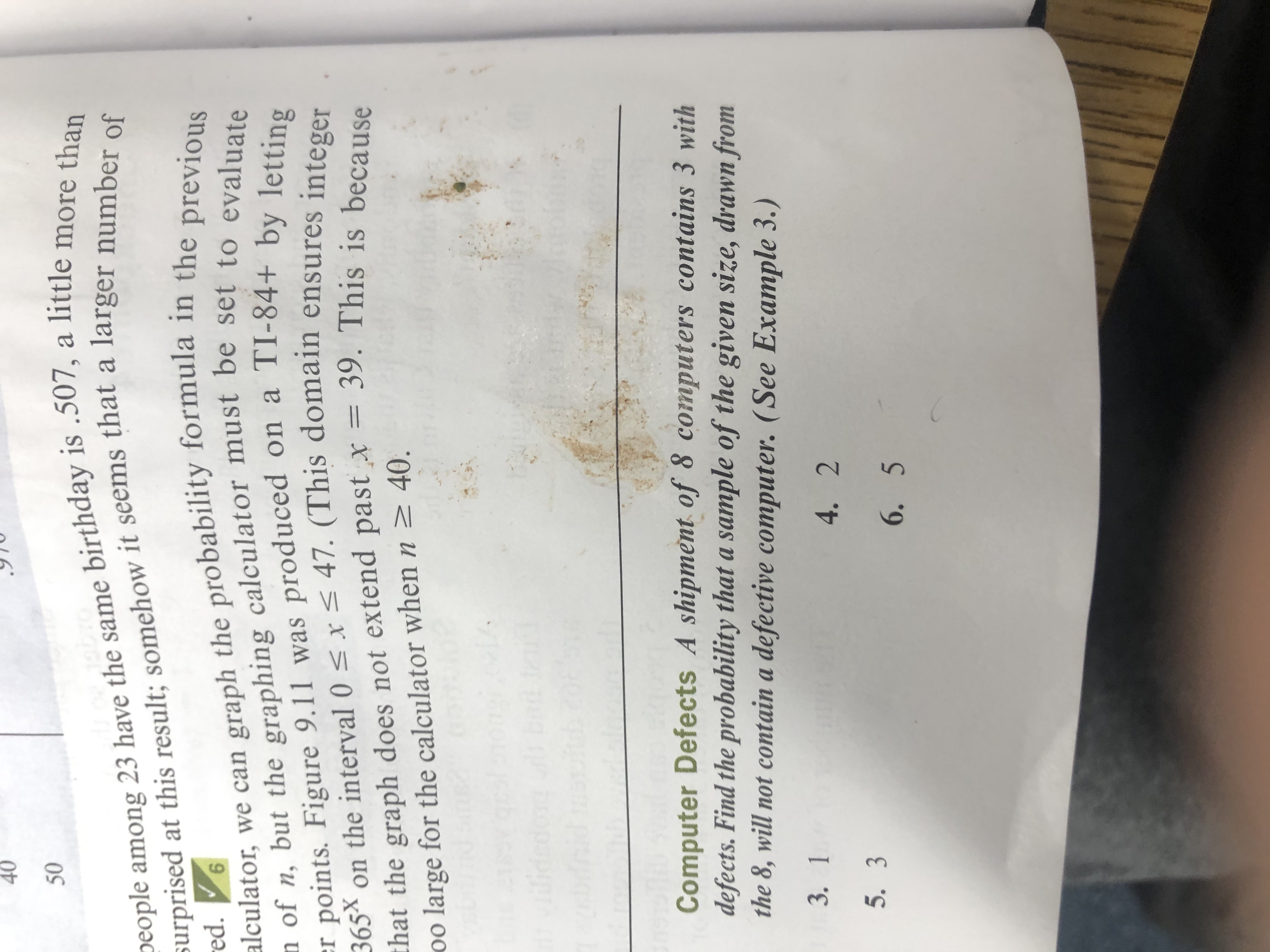# 4050Deople among 23 have the same birthday is .507, a little more thanSurprised at this result; somehow it seems that a larger number ofalculator, we can graph the probability formula in the previousn of n, but the graphing calculator must be set to evaluateer points. Figure 9.11 was produced on a TI-84+ by lettinged.on the interval 0 < x< 47. (This domain ensures integer365Xhat the graph does not extend past x = 39. This is because40.oo large for the calculator when nComputer Defects A shipment of 8 computers contains 3 withdefects. Find the probability that a sample of the given size, drawn fromthe 8, will not contain a defective computer. (See Example 3.)3. 14. 25. 36. 5

Question
1 views

Can u please do number 5help_outlineImage Transcriptionclose40 50 Deople among 23 have the same birthday is .507, a little more than Surprised at this result; somehow it seems that a larger number of alculator, we can graph the probability formula in the previous n of n, but the graphing calculator must be set to evaluate er points. Figure 9.11 was produced on a TI-84+ by letting ed. on the interval 0 < x< 47. (This domain ensures integer 365X hat the graph does not extend past x = 39. This is because 40. oo large for the calculator when n Computer Defects A shipment of 8 computers contains 3 with defects. Find the probability that a sample of the given size, drawn from the 8, will not contain a defective computer. (See Example 3.) 3. 1 4. 2 5. 3 6. 5 fullscreen
check_circle

### Want to see the full answer?

See Solution

#### Want to see this answer and more?

Solutions are written by subject experts who are available 24/7. Questions are typically answered within 1 hour.*

See Solution
*Response times may vary by subject and question.
Tagged in

### Probability